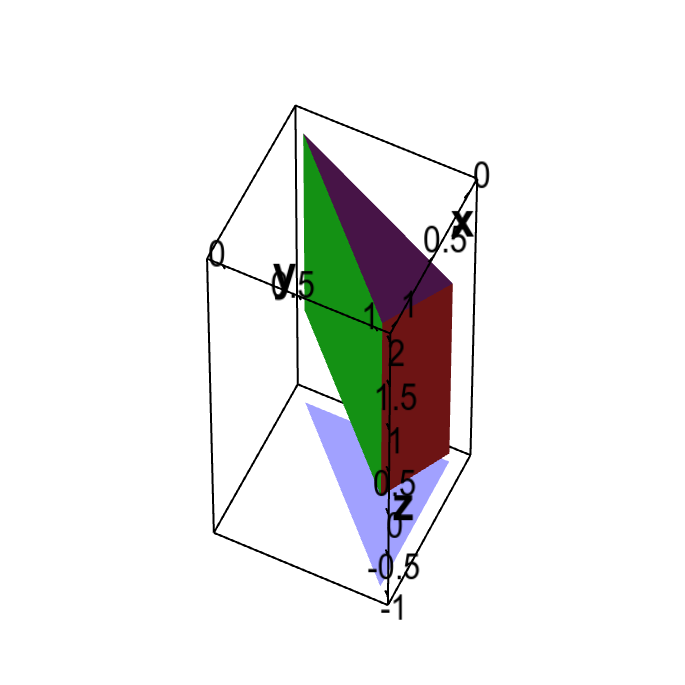# Math Insight

### Applet: Region bounded by planes demonstrating the shadow methodThe region $\dlv$ is bounded by the vertical planes $x=0$, $y=1$, and $x=y$ and the angled planes $z=2+x-y$ and $z=x-y$. If the sun is viewed to be in the direction of the positive $z$-axis, the resulting shadow on a plane perpendicular to the $z$-axis is a triangle, as illustrated by the blue triangle below the region.